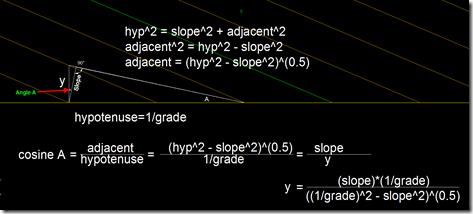## Monday, March 10, 2008

### Formula For Slope

I'm not going to make a judgement on if this is the correct way or the incorrect way to do things, I'm just providing the math.

If you grade from a sloping baseline (be it a feature line or alignment/profile) the grade will be calculated perpendicular to the baseline. This is normal and customary in creating a road corridor; but may not be wanted when creating grading from a featureline. If you measure the distance perpendicular from one contour to the next you will find that the distance isn't an even number (if you use a 2:1 slope) but slightly off. To get the value to be exact use the formula below:This is an Excel formula you can use to calculate the required slope:

=(E2*(1/E1))/(((1/E1)^2-(E2)^2))^0.5

Put the slope (Ex. 2, for a 2:1 slope) in E2 and the grade (in decimal form) in cell E1.